Potential Energy

Conservative forces: A force F is conservative if it can be represented as the gradient of a scalar function. This can written as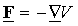where V is a scalar function of position in space. The negative sign is only for convenience so that V can be identified as the potential energy.

Work of a conservative force: Given a conservative force F, its work can be calculated as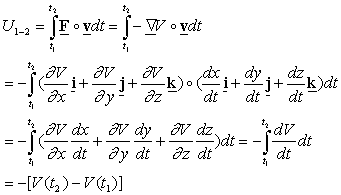Therefore, the work of a conservative force is negative the change in its potential energy.

Note: Since the work of a conservative force does not depend on the path the particle takes when going from its location at time 1 to its location at time 2, the work of a conservative force is path independent.

Example 2

The work-energy relation: The work energy relation can be rewritten to take advantage of the fact that you can calculate the work of conservative forces form the potential energy. Let the resultant work of all forces on a particle be separated into the work done by conservative forces and the work done by non-conservative forces as follows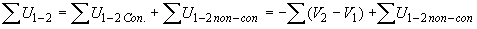Introduction of this into the work energy relation gives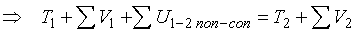Total Mechanical Energy: The total mechanical energy  E is the sum of the kinetic energy and the potential energy of the conservative forces.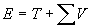The work energy relation can now be written as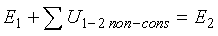Conservative systems: A conservative system is one for which the total mechanical energy E remains constant. This can only happen if the non-conservative forces do no work.

Potential energy of gravity: The potential energy of gravity is given by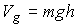where h is measured from an elevation selected as reference.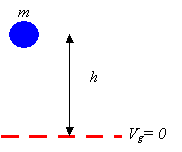Potential energy of a spring: The potential energy of a spring is given by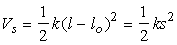where k is the spring stiffness, l is the spring’s current length, and lo is the free length of the spring, and s is the stretch of the spring.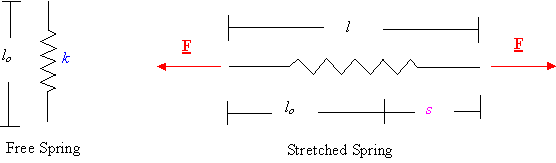Example 3